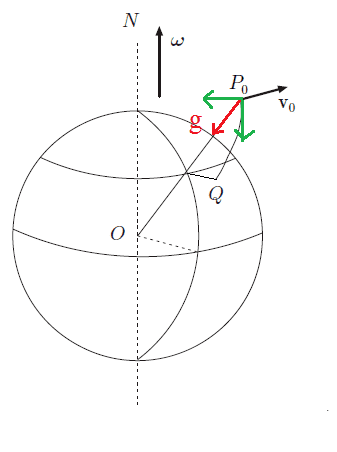# Centrifugal force on Earth and deviation of free falling obj

Centrifugal force due to rotation of the Earth has among its effects the deviation towards the equator of a ball falling from height ##h## vertically on Earth.

I do not understand what is the inertial explanation of this phenomenon. Once the ball is released there is no centripetal force that causes it to curve its path, hence it will move in elliptic trajectory (only due to gravity).

Why does the ball move towards the equator also?

BvU
Homework Helper
Centrifugal force due to rotation of the Earth has among its effects the deviation towards the equator of a ball falling from height ##h## vertically on Earth.
Really ? Do you have a reference or a formula that shows this ?

Once the ball is released there is no centripetal force that causes it to curve its path, hence it will move in elliptic trajectory (only due to gravity).
The earth pulls on the ball. Isn't that a force towards the center of the earth ?

jbriggs444
Homework Helper
If you are considering centrifugal force then trajectories, ellipses, curved paths and Coriolis do not enter in. The relevant question is only on the direction of the net force (gravity plus centrifugal).

The force of gravity points directly at the center of the Earth. Centrifugal force points directly away from the Earth's axis. From the perspective of a person standing on the ground in the northern hemisphere, centrifugal force acts at an angle that is slanted up and south. The vector sum of the two forces is almost vertically down. The contribution from centrifugal force means that it slants slightly south -- toward the equator.

The surface of the earth is itself slanted to be at right angles to the resultant force. The net effect is that the perceived direction of the fall is purely vertical. So, for instance, you will not see the cue ball rolling southward on a pool table because the pool table has been leveled to match the resultant apparent force of gravity.

So actually is gravity that pulls the ball slightly towards the equator?In this drawing I marked in red ##\vec{g}## and in green its component. One of this component is vertical and produces a displacement southwards during the fall. Is that correct?

BvU
Homework Helper
Southwards is misleading: the latitude does not change.
Gravity is the only force acting, indeed. It keeps you in a circular trajectory (so you follow the earth), thus fulfilling the job of centripetal force. The remainder is your weight (the force with which you are pulled down towards the center of the earth). So: on the poles a weight scale will show a higher number for you weight than on the equator ! ( But a balance will require the same mass, whether on pole or on equator )

jbriggs444
Homework Helper
So actually is gravity that pulls the ball slightly towards the equator?
Gravity has a non-zero component toward the plane containing the equator and the centrifugal force does not. So yes, you could say that gravity is the force that produces a displacement toward the equator.

On the other hand, if you consider "south" as pointing toward the equator in the horizontal direction then gravity has a non-zero northward component while centrifugal force has a non-zero southward component and any southward component in the fall direction traces clearly to the centrifugal force.

Edit: Note that details of the above description can vary depending on exactly what you mean by "horizontal".

•Soren4
A.T.